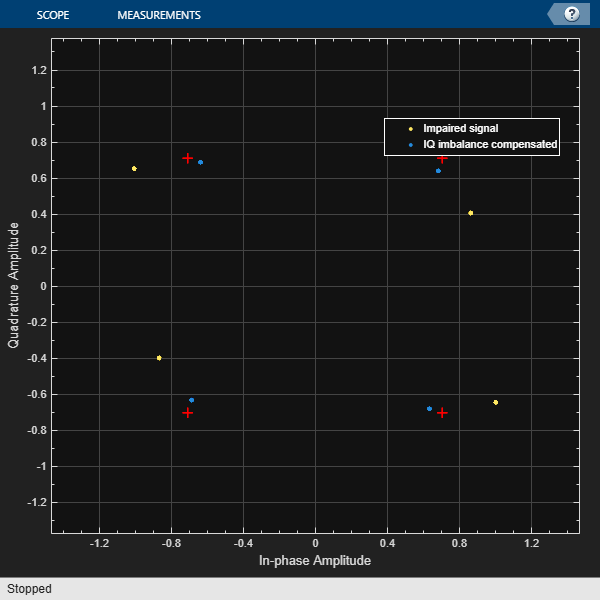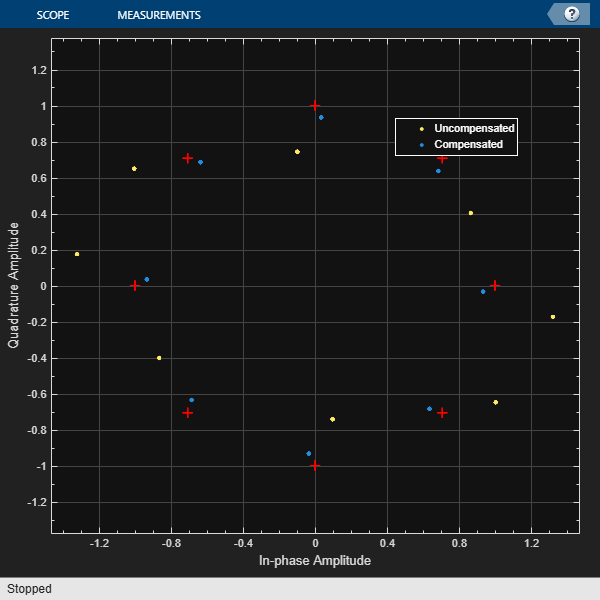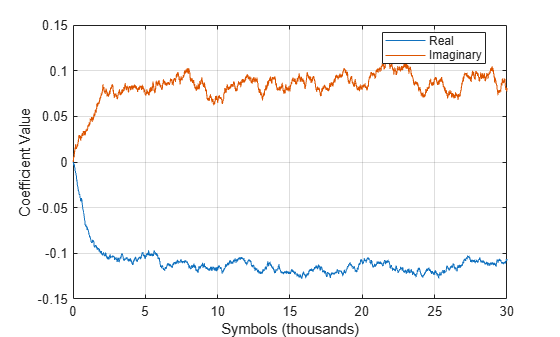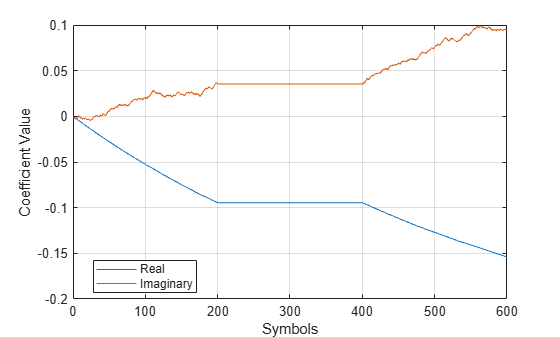Main Content

# comm.IQImbalanceCompensator

Compensate for I/Q imbalance

## Description

The `IQImbalanceCompensator` System object™ compensates for the imbalance between the in-phase and quadrature components of a modulated signal.

To compensate for I/Q imbalance:

1. Define and set up the `IQImbalanceCompensator` object. See Construction.

2. Call `step` to compensate for the I/Q imbalance according to the properties of `comm.IQImbalanceCompensator`. The behavior of `step` is specific to each object in the toolbox.

The adaptive algorithm inherent to the I/Q imbalance compensator is compatible with M-PSK, M-QAM, and OFDM modulation schemes, where M>2.

Note

The output of the compensator might be scaled and rotated, that is, multiplied by a complex number, relative to the reference constellation. In practice, this is not an issue as receivers correct for this prior to demodulation through the use of channel estimation.

Note

Starting in R2016b, instead of using the `step` method to perform the operation defined by the System object™, you can call the object with arguments, as if it were a function. For example, ```y = step(obj,x)``` and `y = obj(x)` perform equivalent operations.

## Construction

`H = comm.IQImbalanceCompensator` creates a compensator System object, `H`, that compensates for the imbalance between the in-phase and quadrature components of the input signal.

`H = comm.IQImbalanceCompensator(Name,Value)` creates an I/Q imbalance compensator object, `H`, with each specified property `Name` set to the specified `Value`. You can specify additional name-value pair arguments in any order as (`Name1`,`Value1`,...,`NameN`,`ValueN`).

## Properties

 `CoefficientSource` Source of compensator coefficients Specify either `Estimated from input signal` or ```Input port```. If the `CoefficientSource` property is set to `Estimated from input signal`, the compensator uses an adaptive algorithm to estimate the compensator coefficient from the input signal. If the `CoefficientSource` property is set to `Input port`, all other properties are disabled and the compensator coefficients must be provided to the `step` function as an input argument. The default value is `Estimated from input signal`. This property is nontunable. `InitialCoefficent` Initial coefficient used to compensate for I/Q imbalance The initial coefficient is a complex scalar that can be either single or double precision. The default value is `0+0i`. This property is nontunable. `StepSizeSource` Source of step size for coefficient adaptation Specify either `Property` or ```Input port```. If `StepSizeSource` is set to `Property`, you specify the step size through the `StepSize` property. Otherwise, the step size is provided to the `step` function as an input argument. The default value is `Property`. This property is nontunable. `StepSize` Adaptation step size Specifies the step size used by the algorithm in estimating the I/Q imbalance. This property is accessible only when `StepSizeSource` is set to `Property`. The default value is `1e-5`. This property is tunable. `AdaptInputPort` Creates input port to control compensator coefficient adaptation When this logical property is `true`, an input port is created to enable or disable coefficient adaptation. If `AdaptInputPort` is `false`, the coefficients update after each output sample. The default value is `false`. This property is nontunable. `CoefficientOutputPort` Create port to output compensator coefficients When this logical property is `true`, the I/Q imbalance compensator coefficients are made available through an output argument of the `step` function. The default value is `false`. This property is nontunable.

## Methods

 step Compensate I/Q Imbalance
Common to All System Objects
`release`

Allow System object property value changes

`reset`

Reset internal states of System object

When using `reset`, this method resets the windowed suffix from the last symbol in the previously processed frame.

## Examples

collapse all

Mitigate the impacts of amplitude and phase imbalance on a QPSK modulated signal by using the comm.IQImbalanceCompensator System object?.

Create a constellation diagram object. Specify name-value pairs to ensure that the constellation diagram displays only the last 100 data symbols.

```constDiagram = comm.ConstellationDiagram(... 'SymbolsToDisplaySource','Property', ... 'SymbolsToDisplay',100); ```

Create an I/Q imbalance compensator.

```iqImbComp = comm.IQImbalanceCompensator; ```

Generate random data symbols and apply QPSK modulation.

```data = randi([0 3],1e7,1); txSig = pskmod(data,4,pi/4); ```

Apply amplitude and phase imbalance to the transmitted signal.

```ampImb = 5; % dB phImb = 15; % deg gainI = 10.^(0.5*ampImb/20); gainQ = 10.^(-0.5*ampImb/20); imbI = real(txSig)*gainI*exp(-0.5i*phImb*pi/180); imbQ = imag(txSig)*gainQ*exp(1i*(pi/2 + 0.5*phImb*pi/180)); rxSig = imbI + imbQ; ```

Plot the constellation diagram of the received signal. Observe that the received signal experienced an amplitude and phase shift.

```constDiagram(rxSig) ```Apply the I/Q compensation algorithm and view the constellation. The compensated signal constellation is nearly aligned with the reference constellation.

```compSig = iqImbComp(rxSig); constDiagram(compSig) ```Compensate for an amplitude and phase imbalance on an 8-PSK signal by using the `comm.IQImbalanceCompensator` System object™ with external coefficients.

Create 8-PSK modulator and constellation diagram System objects. Use name-value pairs to ensure that the constellation diagram displays only the last 100 data symbols and to provide the reference constellation.

```hMod = comm.PSKModulator(8); refC = constellation(hMod); hScope = comm.ConstellationDiagram(... 'SymbolsToDisplaySource','Property', ... 'SymbolsToDisplay',100, ... 'ReferenceConstellation',refC); ```

Create an I/Q imbalance compensator object with an input port for the algorithm coefficients.

```hIQComp = comm.IQImbalanceCompensator('CoefficientSource','Input port'); ```

Generate random data symbols and apply 8-PSK modulation.

```data = randi([0 7],1000,1); txSig = step(hMod,data); ```

Apply amplitude and phase imbalance to the transmitted signal.

```ampImb = 5; % dB phImb = 15; % deg gainI = 10.^(0.5*ampImb/20); gainQ = 10.^(-0.5*ampImb/20); imbI = real(txSig)*gainI*exp(-0.5i*phImb*pi/180); imbQ = imag(txSig)*gainQ*exp(1i*(pi/2 + 0.5*phImb*pi/180)); rxSig = imbI + imbQ; ```

Plot the constellation diagram of the received signal. Observe that the received signal experienced an amplitude and phase shift.

```step(hScope,rxSig); ```Use the `iqimbal2coef` function to determine the compensation coefficient given the amplitude and phase imbalance.

```compCoef = iqimbal2coef(ampImb,phImb); ```

Apply the compensation coefficient to the received signal using the `step` function of the `comm.IQImbalanceCompensator` object and view the resultant constellation. You can see that the compensated signal constellation is now nearly aligned with the reference constellation.

```compSig = step(hIQComp,rxSig,compCoef); step(hScope,compSig) ```Remove an I/Q imbalance from a 64-QAM signal and to make the estimated coefficients externally available while setting the algorithm step size from an input port.

Create a constellation diagram object. Use name-value pairs to ensure that the constellation diagram displays only the last 256 data symbols, set the axes limits, and specify the reference constellation.

```M = 64; refC = qammod(0:M-1,M); constDiagram = comm.ConstellationDiagram(... 'SymbolsToDisplaySource','Property', ... 'SymbolsToDisplay',256, ... 'XLimits',[-10 10],'YLimits',[-10 10], ... 'ReferenceConstellation',refC); ```

Create an I/Q imbalance compensator System object in which the step size is specified as an input argument and the estimated coefficients are made available through an output port.

```iqImbComp = comm.IQImbalanceCompensator('StepSizeSource','Input port', ... 'CoefficientOutputPort',true); ```

Generate random data symbols and apply 64-QAM modulation.

```nSym = 25000; data = randi([0 M-1],nSym,1); txSig = qammod(data,M); ```

Apply amplitude and phase imbalance to the transmitted signal.

```ampImb = 2; % dB phImb = 10; % deg gainI = 10.^(0.5*ampImb/20); gainQ = 10.^(-0.5*ampImb/20); imbI = real(txSig)*gainI*exp(-0.5i*phImb*pi/180); imbQ = imag(txSig)*gainQ*exp(1i*(pi/2 + 0.5*phImb*pi/180)); rxSig = imbI + imbQ; ```

Plot the constellation diagram of the received signal.

```constDiagram(rxSig); ```Specify the step size parameter for the I/Q imbalance compensator.

```stepSize = 1e-5; ```

Compensate for the I/Q imbalance while setting the step size via an input argument. You can see that the compensated signal constellation is now nearly aligned with the reference constellation.

```[compSig,estCoef] = iqImbComp(rxSig,stepSize); constDiagram(compSig) ```Plot the real and imaginary values of the estimated coefficients. You can see that they reach a steady-state solution.

```plot((1:nSym)'/1000,[real(estCoef),imag(estCoef)]) grid xlabel('Symbols (thousands)') ylabel('Coefficient Value') legend('Real','Imag','location','best') ```Control the adaptation algorithm of the I/Q imbalance compensator using an external argument.

Apply QPSK modulation to random data symbols.

```data = randi([0 3],600,1); txSig = pskmod(data,4,pi/4,'gray');```

Create an I/Q imbalance compensator in which the adaptation algorithm is controlled through an input port, the step size is specified through the `StepSize` property, and the estimated coefficients are made available through an output port.

```iqImbComp = comm.IQImbalanceCompensator('AdaptInputPort',true, ... 'StepSize',0.001,'CoefficientOutputPort',true);```

Apply amplitude and phase imbalance to the transmitted signal.

```ampImb = 5; % dB phImb = 15; % deg gainI = 10.^(0.5*ampImb/20); gainQ = 10.^(-0.5*ampImb/20); imbI = real(txSig)*gainI*exp(-0.5i*phImb*pi/180); imbQ = imag(txSig)*gainQ*exp(1i*(pi/2 + 0.5*phImb*pi/180)); rxSig = imbI + imbQ;```

Break the compensation operation into three segments in which the compensator is enabled for the first 200 symbols, disabled for the next 200 symbols, and enabled for the last 200 symbols. Save the coefficient data in three vectors.

```[~,estCoef1] = iqImbComp(rxSig(1:200),true); [~,estCoef2] = iqImbComp(rxSig(201:400),false); [~,estCoef3] = iqImbComp(rxSig(401:600),true);```

Concatenate the complex algorithm coefficients and plot their real and imaginary parts.

```estCoef = [estCoef1; estCoef2; estCoef3]; plot((1:600)',[real(estCoef) imag(estCoef)]) grid xlabel('Symbols') ylabel('Coefficient Value') legend('Real','Imaginary','location','best')```Observe that the coefficients do not adapt during the time in which the compensator is disabled.

## Algorithms

One of the major impairments affecting direct conversion receivers is the imbalance between the received signal’s in-phase and quadrature components. Rather than improving the front-end, analog hardware, it is more cost effective to tolerate a certain level of I/Q imbalance and then implement compensation methods. A circularity-based blind compensation algorithm is used as the basis for the I/Q Imbalance Compensator.

A generalized I/Q imbalance model is shown, where g is the amplitude imbalance and ϕ is the phase imbalance (ideally, g = 1 and ϕ = 0). In the figure, H(f) is the nominal frequency response of the branches due to, for example, lowpass filters. HI(f) and HQ(f) represent the portions of the in-phase and quadrature amplitude and phase responses that differ from the nominal response. With perfect matching, HI(f) = HQ(f) = 1.Let z(t) be the ideal baseband equivalent signal of the received signal, r(t), where its Fourier transform is denoted as Z(f). Given the generalized I/Q imbalance model, the Fourier transform of the imbalanced signal, x(t) = xI(t) + xQ(t), is

`$X\left(f\right)={G}_{1}\left(f\right)Z\left(f\right)+{G}_{2}\left(f\right){Z}^{*}\left(-f\right)$`

where G1(f) and G2(f) are the direct and conjugate components of the I/Q imbalance. These components are defined as

`$\begin{array}{l}{G}_{1}\left(f\right)=\left[{H}_{I}\left(f\right)+{H}_{Q}\left(f\right)g\mathrm{exp}\left(-j\varphi \right)\right]/2\\ {G}_{2}\left(f\right)=\left[{H}_{I}\left(f\right)+{H}_{Q}\left(f\right)g\mathrm{exp}\left(j\varphi \right)\right]/2\end{array}$`

Applying the inverse Fourier transform to X(f), the signal model becomes x(t) = g1(t) * z(t) + g2(t) * z*(t).

This suggests the compensator structure as shown in which discrete-time notation is used to express the variables. The compensated signal is expressed as y(n) = x(n) + wx*(n).A simple algorithm of the form

`$\left\{\begin{array}{l}y\left(n\right)=x\left(n\right)+w\left(n\right){x}^{*}\left(n\right)\\ w\left(n+1\right)=w\left(n\right)-M{y}^{2}\left(n\right)\end{array}$`

is used to determine the weights, because it ensures that the output is “proper”, that is, $E\left[{y}^{2}\left(n\right)\right]=0$ . The initial value of w is determined by the `InitialCoefficient` property, which has a default value of `0 + 0i`. M is the step size, as specified in the `StepSize` property.

## Selected Bibliography

 Anttila, L., M. Valkama, and M. Renfors. “Blind compensation of frequency-selective I/Q imbalances in quadrature radio receivers: Circularity-based approach”, Proc. IEEE ICASSP, pp.III-245–248, 2007.

 Kiayani, A., L. Anttila, Y. Zou, and M. Valkama, “Advanced Receiver Design for Mitigating Multiple RF Impairments in OFDM Systems: Algorithms and RF Measurements”, Journal of Electrical and Computer Engineering, Vol. 2012.

## See Also

Introduced in R2014b

## Support

#### Bridging Wireless Communications Design and Testing with MATLAB

Download white paper